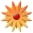Page Views: 2969

Email This Lesson Plan to Me
 Keywords: ratio, scale factor, MATH Subject(s): Algebra, Math Grades 10 through 11 School: Brazosport High School, Freeport, TX Planned By: Nimmy Thomas Original Author: Nimmy Thomas, Freeport
Objectives:
1. Gain a concrete understanding and experience of scale factors and ratios.
2. Understand what a scale factor is.
3. Understand what a ratio is.
4. Understand proportions.
5. Use scale factor and ratios in similar figures to algebraically solve problems.

Students often have difficulty understanding the concepts of scale factors and ratios. A hands-on approach to these topics gives them a more concrete understanding. This foundation will help them jump to the abstract level of applying it to problems. Typical problems where this might be used are the classic height of the tree/flagpole in a to-scale drawing, determining actual distances from a map, determining actual dimensions from a blueprint, etc.

Activity #1 What is a scale factor?
Students take full length photos from a pre-determined distance. Print 4� x 6� photos of these. Students determine the scale factor between the photo and the student�s actual height by trying to find a mathematical relationship between the two. Can you predict another student�s height from their photo using the scale factor you just found?

Activity #2 Is there a ratio?
Use the data from activity 1. Calculate the ratio of each Student�s actual height to the photographic height. Is there any pattern? Can scale factor be thought of as a ratio?
Calculate the ratio of Student A�s height to Student B�s height. Then, calculate the ratio of their photographic heights. Is there any relationship between these ratios?

Activity #3 Does camera-subject distance change the scale factor?
Students take full length photos of each other from 3 different distances. Print photos. Does the scale factor change depending on what the distance is between the camera and the subject?

Activity #4 Can we be photo detectives?
Student groups take photos of objects in the school from a predetermined distance like 5 feet. The groups also record the actual dimensions of the photographed object. After calculating the scale factor on one photo, can the group predict the dimensions of the objects other groups photographed?

Activity #5 Students will create posters and a scrapbook to present findings.
 Materials: Slideshow, Math, Flash/USB Drives, Batteries, Camera Bags, Yearbook, Point and Shoot, Mobile Labs